Last week I’ve presented the PL/SQL Cop tool suite to a customer in Germany. While preparing the demo I had taken my first deeper look at the PL/SQL Cop SonarQube plugin, written by Peter Rohner, a fellow Trivadian. I was impressed how well the additional PL/SQL Cop metrics integrate into SonarQube and how easy it is to monitor the code evolution.

Before I show the code evolution I will go through the metric definitions based on fairly simple example. If you are not interested in the math, then feel free to skip reading the metric sections.

As a starting point I use the following simplified password verification procedure, which ensures that every password contains a digit. I know this procedure is not a candidate for “good PL/SQL code”, but nonetheless it is based on a real live example. The goal of this piece of code is to explain some metrics, before starting to improve the code.

After running this code through PL/SQL Cop I get the following metrics. I show here just the SonarQube output, but the results are the same for the command line utility and the SQL Developer extension of SQL Cop.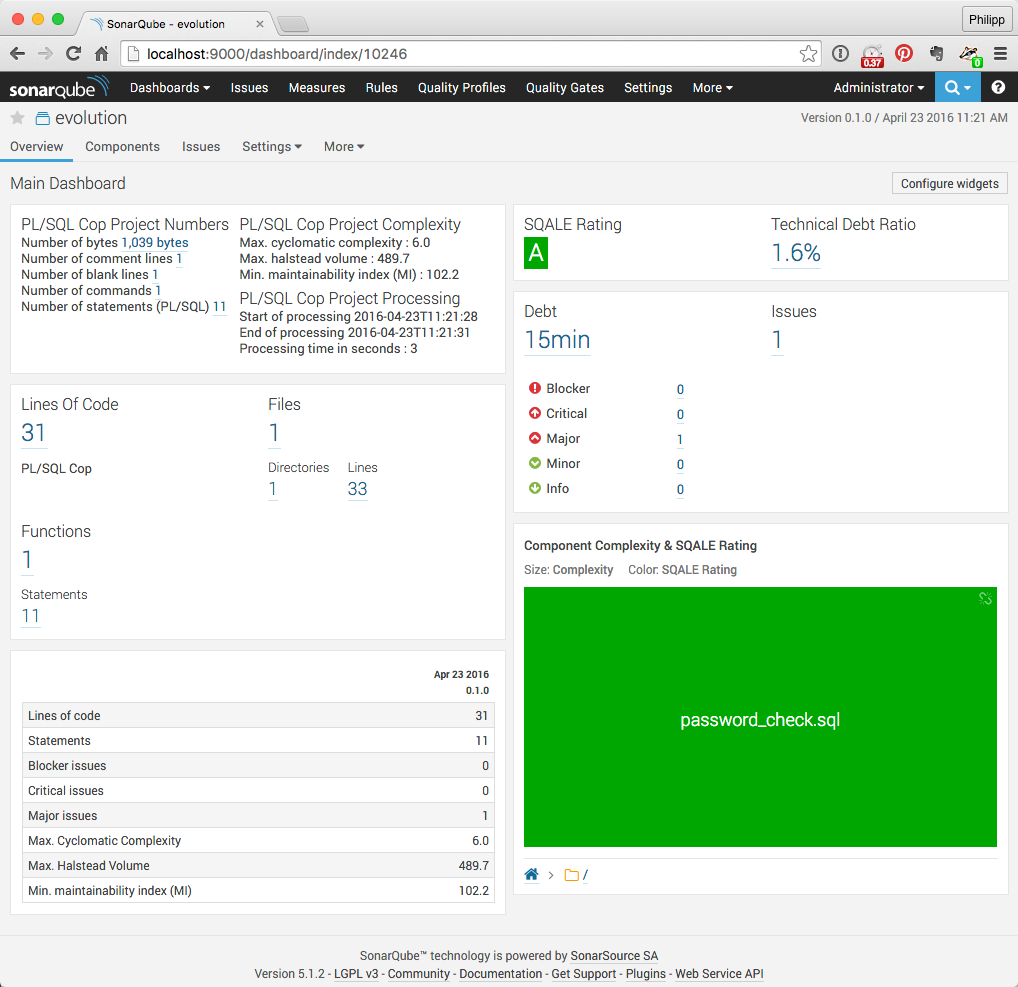Simple Metrics

Here are the definitions of the simple metrics shown above.

• Bytes – the number of bytes (1039)
• Lines – the number of physical lines – lines separated by OS specific line separator (33)
• Comment Lines – the number of comment lines – see line 10 (1)
• Blank Lines – the number of empty lines – see line 28 (1)
• Lines Of Code  – Lines minus comment lines minus blank lines (31)
• Commands – the number of commands from a SQL*Plus point of view – see CREATE OR REPLACE PROCEDURE (1)
• Functions – the number of program units – the password_check procedure (1)
• Statements – the number of PL/SQL statements – 4 assignments, 2 FOR loops, 2 IF statements, 1 GOTO statement, 1 NULL statement, 1 procedure call (11)
• Files – the number of files processed (1)
• Directories – the number of directories processed (1)
• Issues – the number of Trivadis PL/SQL & SQL Coding Guideline violations – Guideline 39: Never use GOTO statements in your code (1)

Simple metrics such as Lines of Code are an easy way to categorise the programs in a project. But the program with the most lines of code does not necessarily have to be the most complex one. Other metrics are better suited to identify the complex parts of a project. But it is important to have a good idea how such metrics are calculated, because no single metric is perfect. I typically identify programs to have a closer look at by a combination of metrics such as lines of code, statements, cyclomatic complexity and the number of severe issues.

See SonarQube documentation for the further metric definitions. Please note, that PL/SQL Cop does not calculate all metrics and some metrics are calculated a bit differently, e.g. Comment Lines.

SQALE Rating

SonarQube rates a project using the SQALE Rating which is is based on the Technical Dept Ratio and calculated as follows:

$$\text'tdr' = 100⋅{\text'Technical Debt'}/{\text'Development Cost'}$$

where

• $\text'tdr'$ is defined as the technical dept ratio (1.6%)
• $\text'Technical Debt'$ is defined as the estimated time to fix the issues, PL/SQL Cop defines the time to fix per issue type (0.25 hours)
• $\text'Development Cost'$ is defined as the estimated time to develop the source code from scratch, the SonarQube default configuration is 30 minutes per Line of Code, you may amend the value on the Technical Dept page (15.5 hours)

The ranges for the SQALE Rating values A (very good) to E (very bad) are based on the SQALE Method Definition Document. SonarQube uses the default rating scheme “0.1,0.2,0.5,1” which may be amended on the Technical Debt page. The rating scheme defines the rating thresholds for A, B, C and D. Higher values lead to an E rating. Here is another way to represent the default rating scheme:

• A: $\text'tdr'$ <= 10%
• B: $\text'tdr'$ > 10% and $\text'tdr'$ <= 20%
• C: $\text'tdr'$ > 20% and $\text'tdr'$ <= 50%
• D: $\text'tdr'$ > 50% and $\text'tdr'$ <= 100%
• E: $\text'tdr'$ > 100%

Based on the default SQALE Rating scheme, a project rated as “E” should be rewritten from scratch, since it would take more time to fix all issues.

McCabe’s Cyclomatic Complexity

Thomas J. McCabe introduced 1976 the metric Cyclomatic Complexity which counts the number of paths in the source code. SonarQube uses this metric to represent the complexity of a program. PL/SQL Cop calculates the cyclomatic complexity as follows:

$$M=E-N+2P$$

where

• $M$ is defined as the cyclomatic complexity (6)
• $E$ is defined as the number of edges (15)
• $N$ is defined as the number of nodes (11)
• $P$ is defined as the number of connected components/programs (1)

The higher the cyclomatic complexity, the more difficult it is to maintain the code.

Please note that PL/SQL Cop V1.0.16 adds an additional edge for ELSE branches in IF/CASE statements, for PL/SQL blocks and for GOTO statements. I consider this a bug. However, Toad Code Analysis (Xpert) calculates the Cyclomatic Complexity the very same way.

PL/SQL Cop calculates the Cyclomatic Complexity per program unit and provides the aggregated Max. Cyclomatic Complexity on file level.

Maurice H. Halstead introduced 1977 the metric Halstead Volume which defines the complexity based on the vocabulary and the total number of words/elements used within a program. In his work Halstead showed also how to express the complexity of academic abstracts using his metrics. PL/SQL Cop calculates the Halstead volume as follows:

$$V=N⋅log_2n$$

where

• $V$ is defined as the Halstead Volume. (489.7)
• $N$ is defined as the program length. $N=N_1+N_2$ (94)
• $n$ is defined as the program vocabulary. $n=n_1+n_2$ (37)
• $N_1$ is defined as the total number of operators (42)
• $N_2$ is defined as the total number of operands (52)
• $n_1$ is defined as the number of distinct operators (11)
• $n_2$ is defined as the number of distinct operands (26)

using

• the following operators: if, then, elsif, case, when, else, loop, for-loop, forall-loop, while-loop, exit, exit-when, goto, return, close, fetch, open, open-for, open-for-using, pragma, exception, procedure-call, assignment, function-call, sub-block, parenthesis, and, or, not, eq, ne, gt, lt, ge, le, semicolon, comma, colon, dot, like, between, minus, plus, star, slash, percent
• the following operands: identifier, string, number

The higher the Halstead volume, the more difficult it is to maintain the code.

PL/SQL Cop calculates the Halstead Volume per program unit and provides the aggregated Max. Halstead Volume on file level.

Maintainability Index

Paul Oman and Jack Hagemeister introduced 1991 the metric Maintainability Index which weighs comments and combines it with Halstead Volume and Cyclomatic Complexity. PL/SQL Cop calculates the maintainability index as follows:

$$\text'MI'=\text'MI'woc+\text'MI'cw$$

where

• $\text'MI'$ is defined as the Maintainablity Index (102.2)
• $\text'MI'woc$ is defined as the $\text'MI'$ without comments. $\text'MI'woc=171−5.2⋅log_eaveV−0.23⋅aveM−16.2⋅log_eaveLOC$ (86.617)
• $\text'MI'cw$ is defined as the $\text'MI'$ comment weight. $\text'MI'cw=50⋅sin(√{{2.4⋅aveC}/{aveLOC}})$ (15.549)
• $aveV$ is defined as the average Halstead volume. $aveV={∑unitLOC⋅V}/{fileLOC}$ (489.7)
• $aveM$ is defined as the average cyclomatic complexity. $aveM={∑unitLOC⋅M}/{fileLOC}$ (6)
• $aveLOC$ is defined as the average lines of code including comments. $aveLOC={∑unitLOC}/{units}$ (24)
• $aveC$ is defined as the average lines of comment. $aveC={∑unitC}/{units}$ (1)
• $unitLOC$ is defined as the number of lines in a PL/SQL unit, without declare section (24)
• $fileLOC$ is defined as the number of lines in source file (33)
• $units$ is defined as the number of PL/SQL units in a file (1)
• $unitC$ is defined as the number of comment lines in a PL/SQL unit (1)

The lower the maintainability index, the more difficult it is to maintain the code.

PL/SQL Cop calculates the Maintainability Index per program unit and provides the aggregated Min. Maintainability Index on file level.

To get rid of the GOTO I’ve rewritten the procedure to use regular expressions to look for digits within the password. The code looks now as follows:

After loading the new version into SonarQube, the dashboard looks as follows: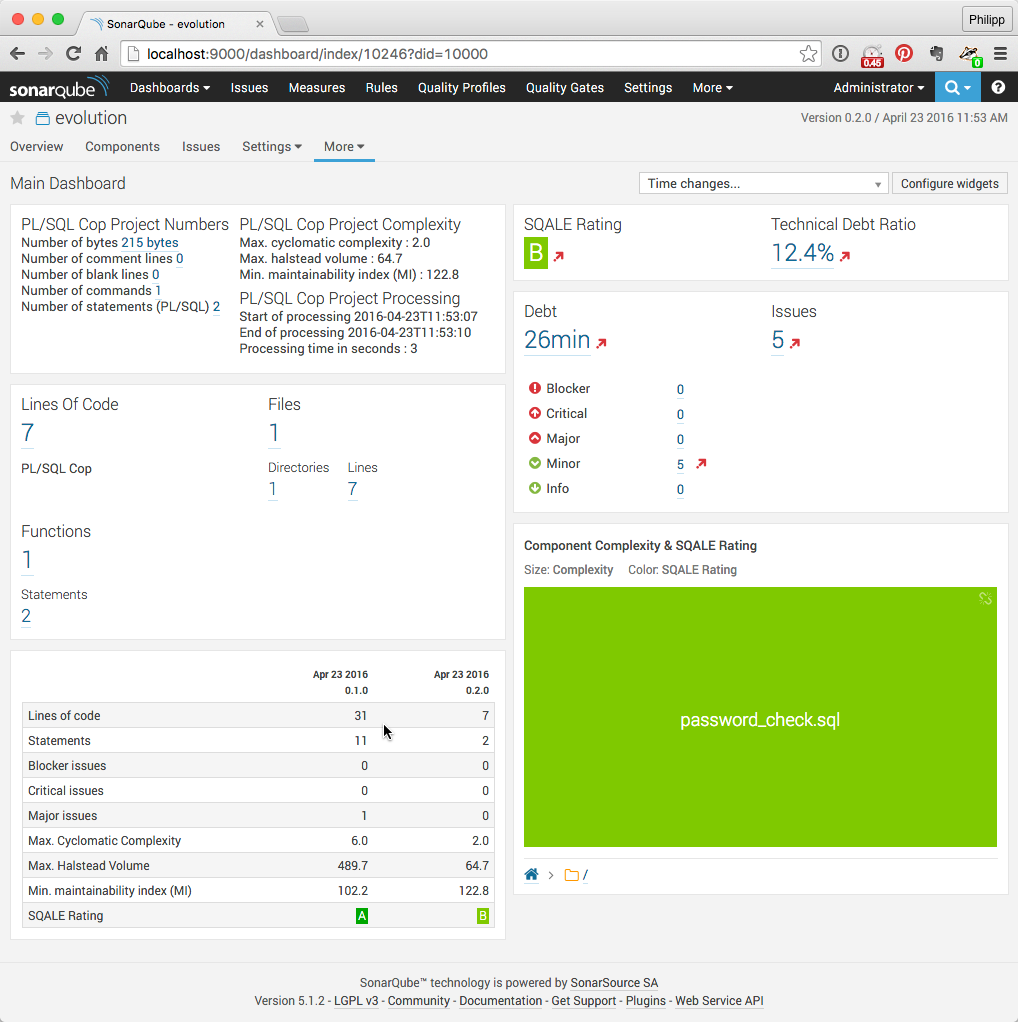Almost all metrics look better now. But instead of 1 major issue I have now 5 minor ones. This leads to a higher Technical Dept Ratio and a bad trend in this area. So let’s see what these minor issues are.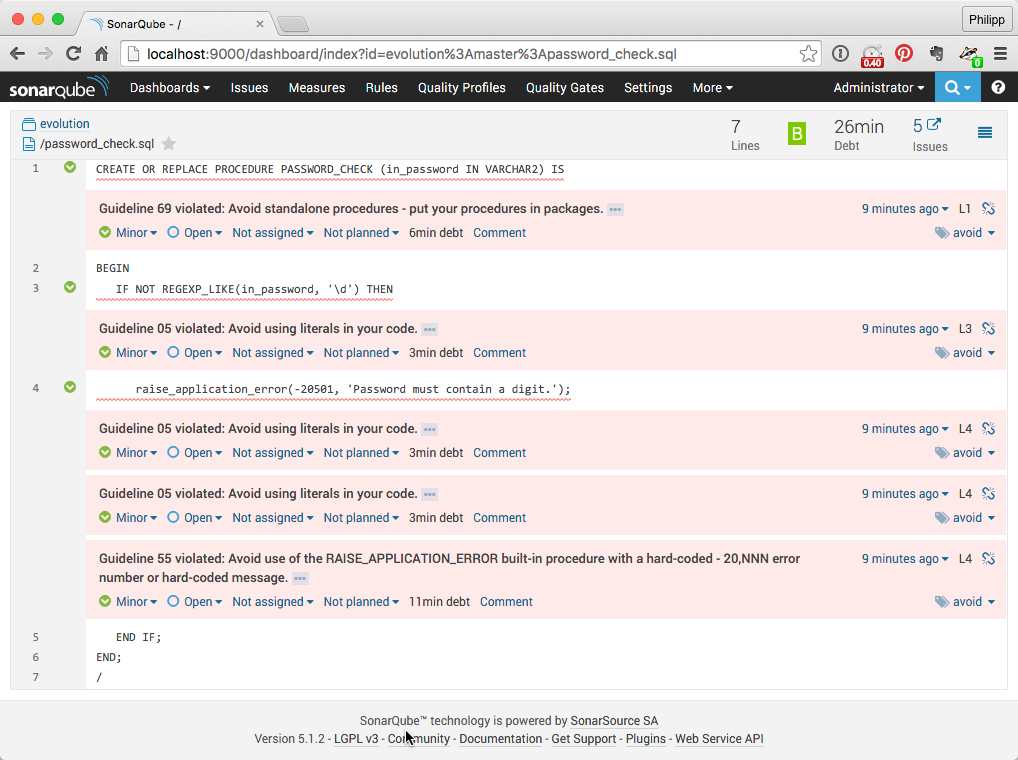I consider all guideline violations as not worth to fix and marked them as “won’t fix”. After reloading the unchanged password_check.sql the SonarQube dashboard looks as follows: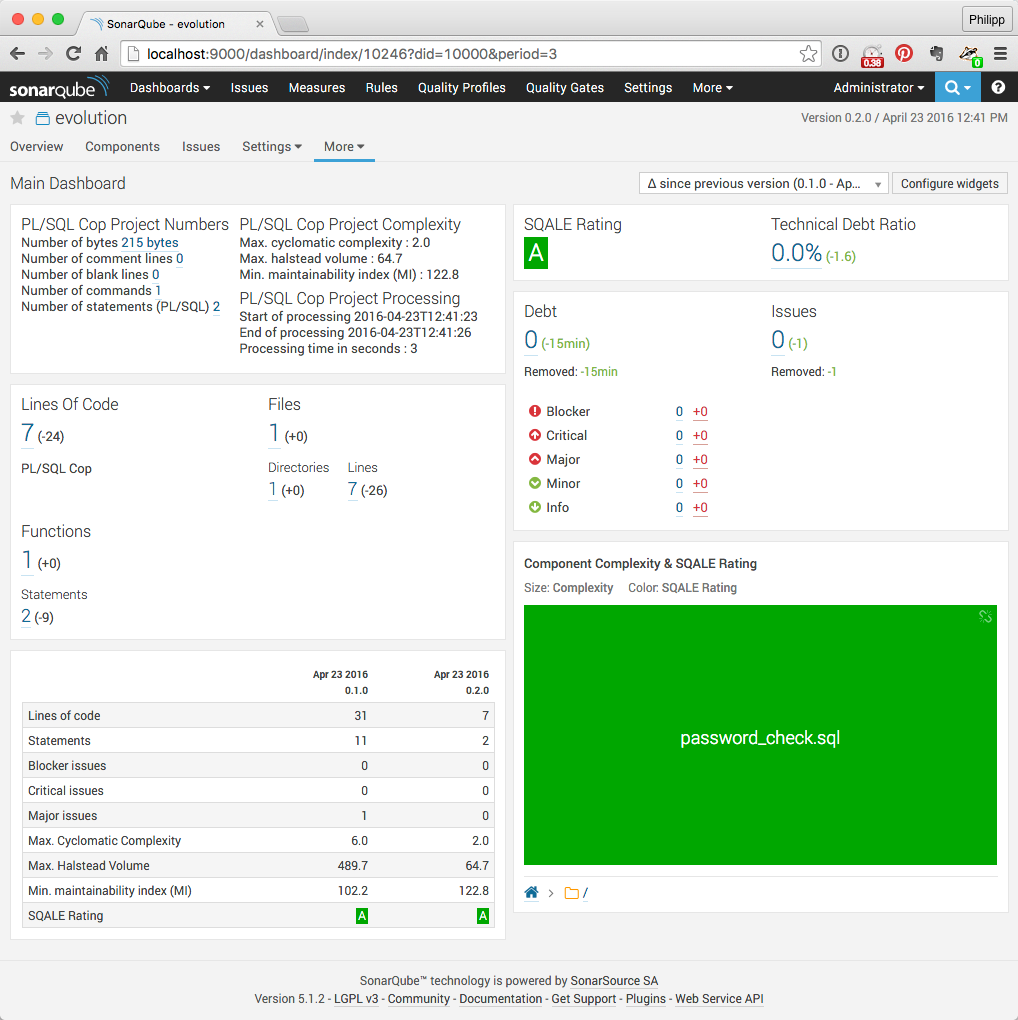The differences/improvement to the previous version is shown in parenthesis.

Password_Check, Version 0.3 – Even Better?

The version 0.2 code looks really good. No technical debt, no issues. A complexity of 2 and just 7 lines of code. But is it possible to improve this code further? Technically yes, especially since we know how the Maintainability Index is calculated. We could simply reduce the Lines of Code as follows:

And after loading the new version into SonarQube the dashboard looks as follows:Reducing the number of lines from 7 to 2 leads to better Maintainability Index, but the number of statements, the Cyclomatic Complexity and Halstead Volume are still the same. The change from version 0.2 to 0.3 reduces the readability of the code and has a negative value. That clearly shows, that the Maintainability Index has its flaws (see also https://avandeursen.com/2014/08/29/think-twice-before-using-the-maintainability-index/). There are various ways to discourage such kind of changes in a project. Using a formatter/beautifier with agreed settings is my favourite.

Code Evolution

SonarQube shows the metrics of the latest two versions in the dashboard. Use the Time Machine page to show metrics of more than two versions of project.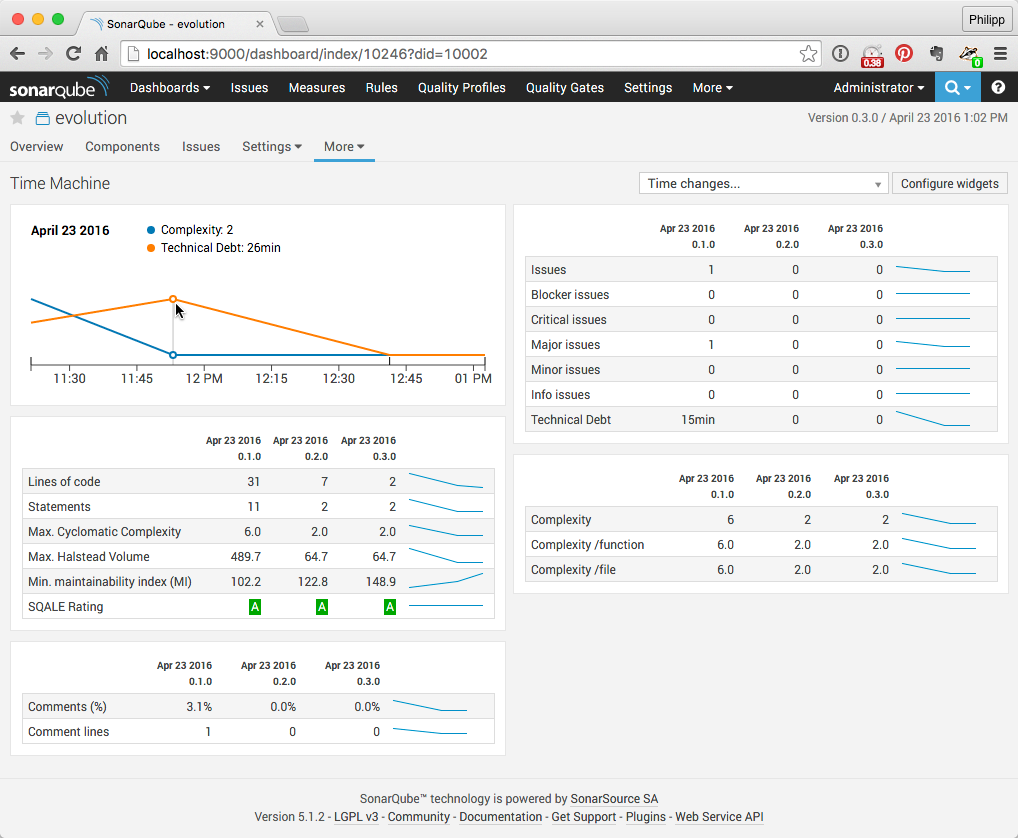Or use the Compare page to compare metrics between versions or projects.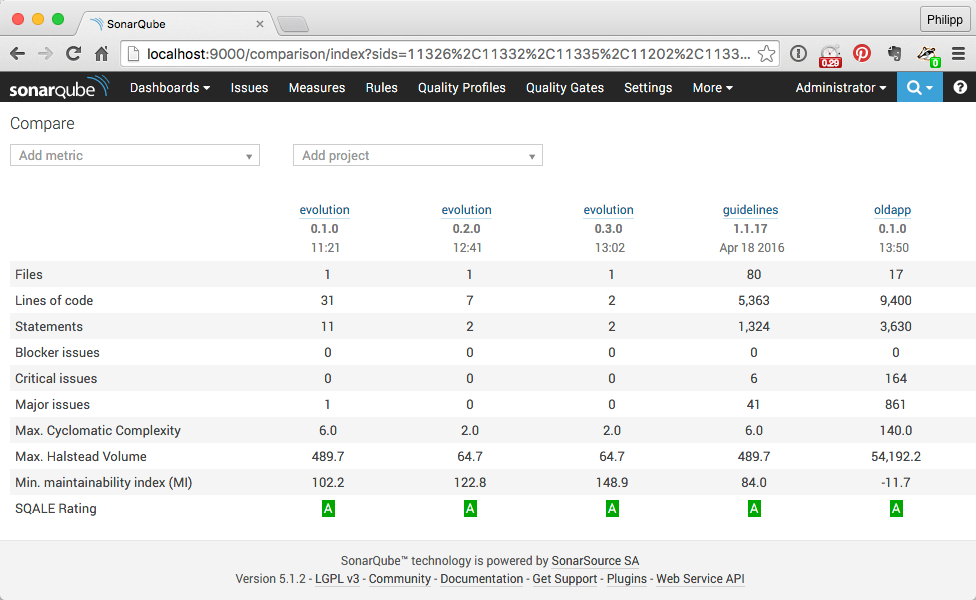Conclusion

Every metric has its flaws, for example

• Lines of Code does not account for the code complexity
• Cyclomatic Complexity does not account for the length of a program and the complexity of a statement
• Halstead Volume does not account for the number of paths in the program
• Maintainability index cannot distinguish between useful and useless comments and does not account for code formatting

But these metrics are still useful to identify complex programs, to measure code evolution (improvements, degradations) and to help you writing better PL/SQL, if you do not trust in metrics blindly.

This site uses Akismet to reduce spam. Learn how your comment data is processed.